### Home > PC > Chapter 2 > Lesson 2.3.5 > Problem2-133

2-133.
1. Square ABCD of area 9 cm2 has the largest possible equilateral triangle inscribed in it. The triangle has vertices A, E, and F, where E is between B and C, and F is between C and D. Homework Help ✎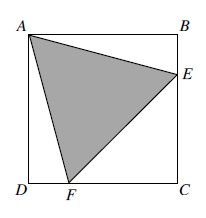1. Why is ΔADF ≅ ΔABE?

2. Why is ΔCEF isosceles?

3. Find the area of triangle AEF.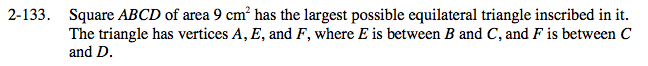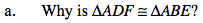In answering these questions, think about the degree measures of the angles.
Label the interior angles of the equilateral triangle.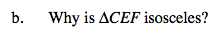Find and label the base angles of the isosceles triangle.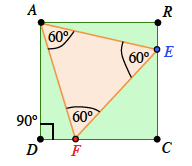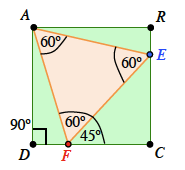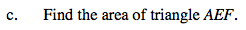Find the angles in triangle AEF. Then use a trig function to find a side of the equilateral triangle.
Then find the height of the equilateral triangle. At that point, you can use the formula: .5(base)(height).